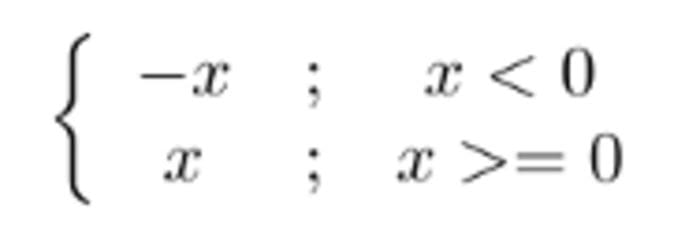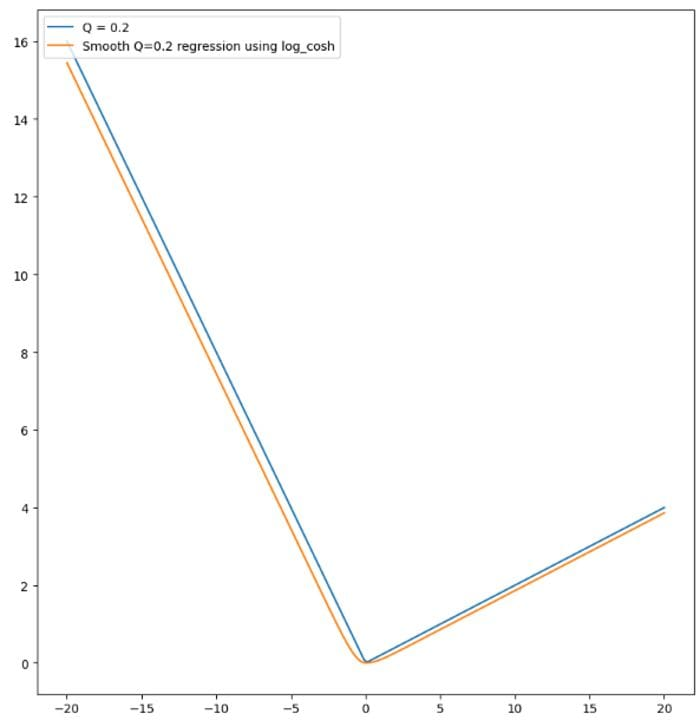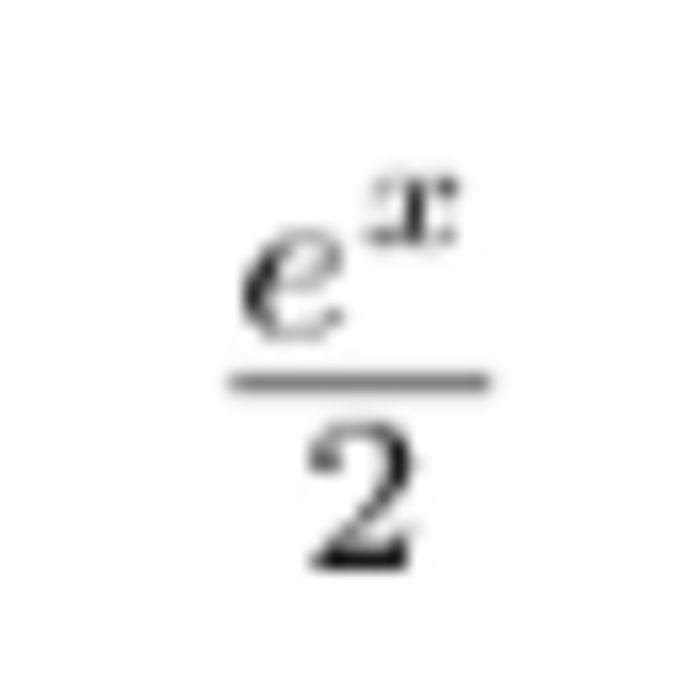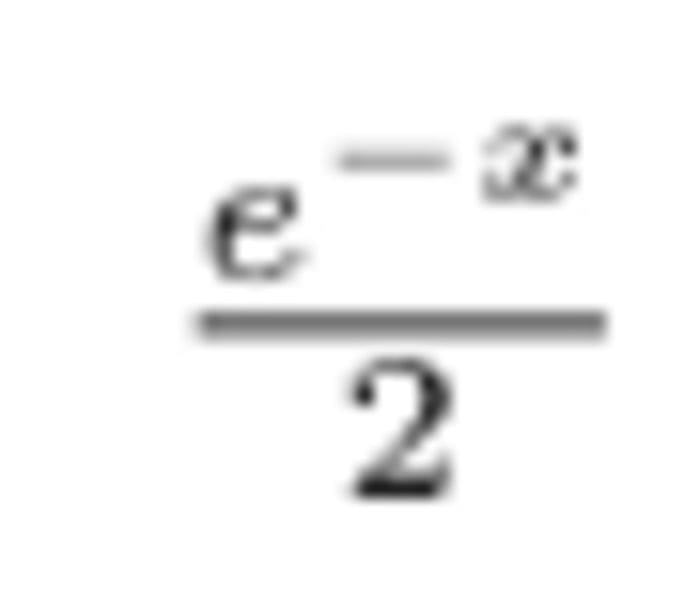# Confidence Intervals for XGBoost

By Guillaume Saupin, CTO at Verteego

Gradient Boosting methods are a very powerful tool for performing accurate predictions quickly, on large datasets, for complex variables that depend non linearly on a lot of features. The underlying mathematical principles are explained with code here.

Moreover, it has been implemented in various ways: XGBoostCatBoostGradientBoostingRegressor, each having its own advantages, discussed here or here. Something these implementations all share is the ability to choose a given objective for training to minimize. And even more interesting is the fact that XGBoost and CatBoost offer easy support for a custom objective function.

### Why do I need a custom objective?

Most implementations provide standard objective functions, like Least Square, Least Deviation, Huber, RMSE, … But sometimes, the problem you’re working on requires a more specific solution to achieve the expected level of precision. Using a custom objective is usually my favourite option for tuning models.

### Can you provide us with an example?

Sure! Recently, I’ve been looking for a way to associate the prediction of one of our models with confidence intervals. As a short reminder, confidence intervals are characterised by two elements:

1. An interval [x_l, x_u]
2. The confidence level C that ensures that C% of the time, the value that we want to predict will lie in this interval.

For instance, we can say that the 99% confidence interval of average temperature on earth is [-80, 60].

Associating confidence intervals with predictions allows us to quantify the level of trust in a prediction.

### How do you compute confidence intervals?

You’ll need to train two models :

• One for the upper bound of your interval
• One for the lower bound of your interval

And guess what? You need specific metrics to achieve that: Quantile Regression objectives. Both the scikit-learn GradientBoostingRegressor and CatBoost implementations provide a way to compute these, using Quantile Regression objective functions, but both use the non-smooth standard definition of this regression :Where t_i is the ith true value and a_i is the ith predicted value. w_i are optional weights used to ponderate the error. And alpha defines the quantile.

For instance, using this objective function, if you set alpha to 0.95, 95% of the obervations are below the predicted value. Conversely, if you set alpha to 0.05, only 5% of the observations are below the prediction. And 90% of real values lie between these two predictions.

Let’s plot it using the following code, for the range [-10, 10] and various alphas:

As you can see in the resulting plot below, this objective function is continuous but its derivative is not. There is a singularity in (0, 0), i.e. it’s a C_0 function, with respect to the error, but not a C_1 function. This is an issue, as gradient boosting methods require an objective function of class C_2, i.e. that can be differentiated twice to compute the gradient and hessian matrices.If you are familiar with the MAE objective, you should have recognized that these quantile regression functions are simply the MAE, scaled and rotated. If you’re not, the screenshot below should convince you :### The logcosh objective

As a reminder, the formula for the MAE objective is simplyMAE objective formula

The figure above also shows a regularized version of the MAE, the logcosh objective. As you can see, this objective is very close to the MAE, but is smooth, i.e. its derivative is continuous and differentiable. Hence, it can be used as an objective in any gradient boosting method, and provides a reasonable rate of convergence compared to default, non-differentiable ones.

And as it is a very close approximation of the MAE, if we manage to scale and rotate it, we’ll get a twice differentiable approximation of the quantile regression objective function.

You might have noticed that there is a slight offset between the curve of the MAE and the log cosh. We will explain that in detail a little further below.

The formula for the logcosh is straightforward :Formula for the logcosh objective

### Rotation and scaling of the logcosh

All we need to do now is to find a way to rotate and scale this objective so that it becomes a good approximation of the quantile regression objective. Nothing complex here. As logcosh is similar to the MAE, we apply the same kind of change as for the Quantile Regression, i.e. we scale it using alpha :Smooth Quantile regression using log cosh

That can be done with these twelve lines of code:

And this works, as shown below :### But wait a minute!

You might be curious as to why combining two non-linear functions like log and cosh results in such a simple, near linear curve.

The answer lies in the formula of cosh :cosh formula

When x is positive and large enough, cosh can be approximated byApproximation of cosh when x >> 0

Conversely, when x is negative enough, cosh can be approximated byApproximation of cosh when x << 0

We begin to understand how combining these two formulae leads to such linear results. Indeed, as we apply the log to these approximations of cosh, we get :logcosh simplification for x >> 0

for x >>0. The same stands for x << 0 :It is now clear why these two functions closely approximate the MAE. We also get as a side benefit the explanation for the slight gap between the MAE and the logcosh. It’s log(2)!

### Let’s try it on a real example

It is now time to ensure that all the theoretical maths we perform above works in real life. We won’t evaluate our method on a simple sinus, as proposed in scikit here ;) Instead, we are going to use real-world data, extracted from the TLC trip record dataset, that contains more than 1 billion taxi trips.

The code snippet below implements the idea presented above. It defines the logcosh quantile regression objective log_cosh_quantile, that computes its gradient and the hessian. Those are required to minimize the objective.

As stated at the beginning of this article, we need to train two models, one for the upper bound, and another one for the lower bound.

The remaining part of the code simply loads data and performs minimal data cleaning, mainly removing outliers.

In this code, we have chosen to compute the 90% confidence interval. Hence we use alpha=0.95 for the upper bound, and alpha=0.05 for the lower bound.

Hyperparameter tuning has been done manually, using fairly standard values. It could certainly be improved, but the results are good enough to illustrate this paper.

The last lines of the script are dedicated to the plotting of the first 150 predictions of the randomly build test set with their confidence interval:Note that we have also included at the end of the script a counter to evaluate the number of real values whose confidence interval is correct. On our test set, 22 238 over 24 889 (89.3%) of the real values were within the calculated confidence interval.

The model has been trained on the first 100 000 lines of the January 2020 dataset of the TLC trip record dataset.

### Conclusion

With simple maths, we have been able to define a smooth quantile regression objective function, that can be plugged into any machine learning algorithm based on objective optimisation.

Using these regularized functions, we have been able to predict reliable confidence intervals for our prediction.

This method has the advantage over the one presented here of being parameters-less. Hyperparameter tuning is already a demanding step in optimizing ML models, we don’t need to increase the size of the configuration space with another parameter ;)

Bio: Guillaume Saupin is CTO at Verteego.

Original. Reposted with permission.

Related: